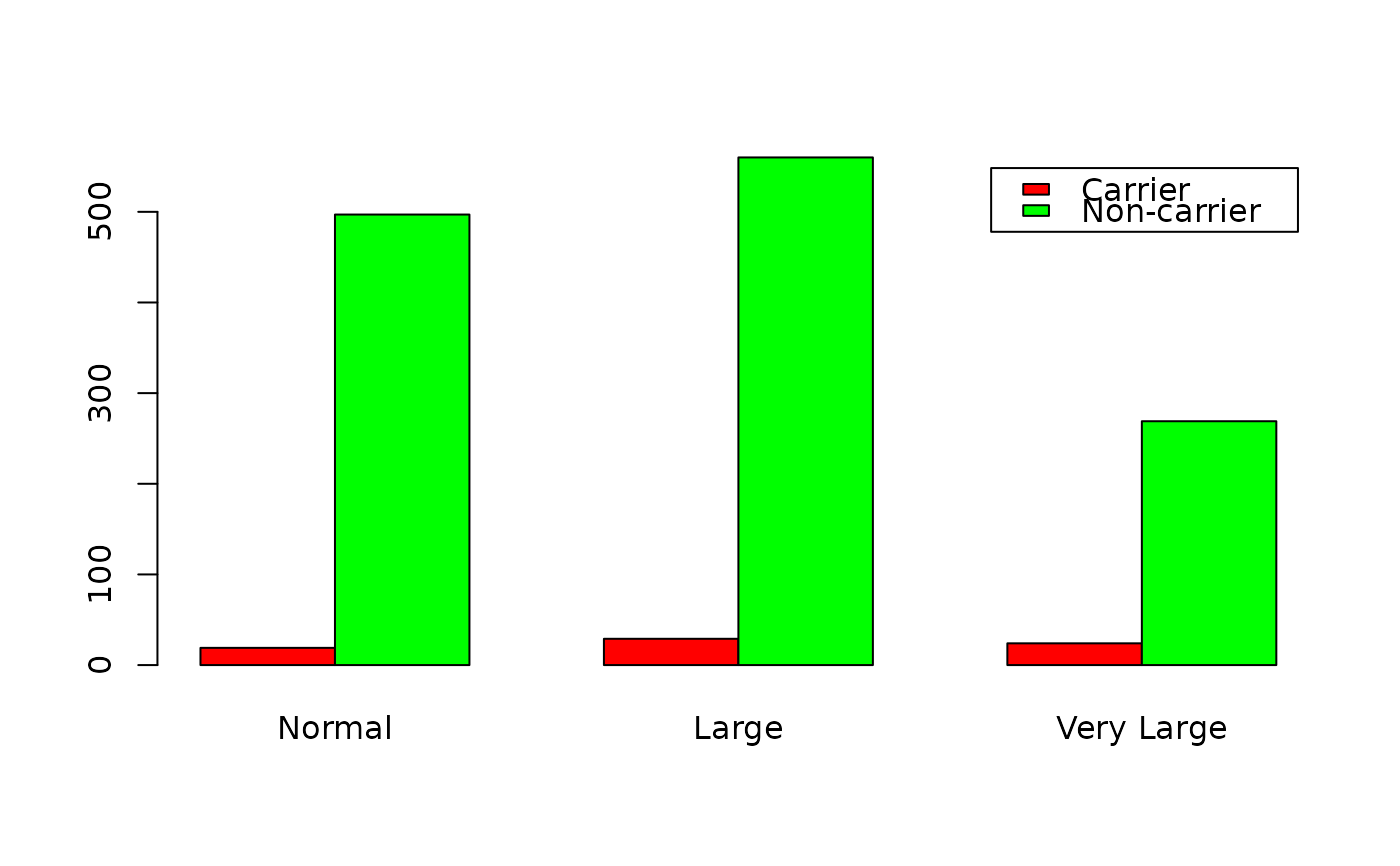Data for Exercise 2.78

Tonsils

## Format

A data frame/tibble with 1,398 observations on two variables

size

a factor with levels Normal, Large, and Very Large

status

a factor with levels Carrier and Non-carrier

## Examples


T1 <- xtabs(~size + status, data = Tonsils)
T1
#>             status
#> size         Carrier Non-carrier
#>   Normal          19         497
#>   Large           29         560
#>   Very Large      24         269
prop.table(T1, 1)
#>             status
#> size            Carrier Non-carrier
#>   Normal     0.03682171  0.96317829
#>   Large      0.04923599  0.95076401
#>   Very Large 0.08191126  0.91808874
prop.table(T1, 1)[2, 1]
#>  0.04923599
barplot(t(T1), legend = TRUE, beside = TRUE, col = c("red", "green"))if (FALSE) {
library(dplyr)
library(ggplot2)
NDF <- dplyr::count(Tonsils, size, status)
ggplot2::ggplot(data = NDF, aes(x = size, y = n, fill = status)) +
geom_bar(stat = "identity", position = "dodge") +
scale_fill_manual(values = c("red", "green")) +
theme_bw()
}